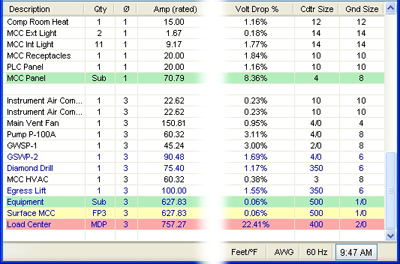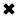# Voltage drop formula for a given cable size#### Use a voltage drop formula to assist you to manually calculate voltage drop in cables

To accurately calculate the voltage drop for a given cable size, length, and current, you need to accurately know the resistance of the type of cable you’re using. Voltage drop formulas can assist you to manually calculate voltage drop in cables that are under full load in branch circuits. It does not matter if you are working with copper or aluminum conductors.

DC / single phase calculation

The voltage drop V in volts (V) is equal to the wire current I in amps (A) times 2 times one way wire size length L in feet (ft) times the wire resistance per 1000 feet R in ohms (Ω/kft) divided by 1000:

Vdrop (V) = Iwire (A) × Rwire(Ω)

Iwire (A) × (2 × L(ft) × Rwire(Ω/kft) / 1000(ft/kft))

The voltage drop V in volts (V) is equal to the wire current I in amps (A) times 2 times one way wire length L in meters (m) times the wire resistance per 1000 meters R in ohms (Ω/km) divided by 1000:

Vdrop (V) = Iwire (A) × Rwire(Ω)

Iwire (A) × (2 × L(m) × Rwire (Ω/km) / 1000(m/km))

3 phase calculation

The line to line voltage drop V in volts (V) is equal to square root of 3 times the wire current I in amps (A) times one way wire length L in feet (ft) times the wire resistance per 1000 feet R in ohms (Ω/kft) divided by 1000:

Vdrop (V) = √3 × Iwire (A) × Rwire (Ω)

= 1.732 × Iwire (A) × (L(ft) × Rwire (Ω/kft) / 1000(ft/kft))

The line to line voltage drop V in volts (V) is equal to square root of 3 times the wire current I in amps (A) times one way wire length L in meters (m) times the wire resistance per 1000 meters R in ohms (Ω/km) divided by 1000:

Vdrop (V) = √3 × Iwire (A) × Rwire (Ω)

= 1.732 × Iwire (A) × (L(m) × Rwire (Ω/km) / 1000(m/km))

Wire diameter calculations

The n gauge wire diameter dn in inches (in) is equal to 0.005in times 92 raised to the power of 36 minus gauge number n, divided by 39:

dn (in) = 0.005 in × 92(36-n)/39

The n gauge wire diameter dn in millimeters (mm) is equal to 0.127mm times 92 raised to the power of 36 minus gauge number n, divided by 39:

dn (mm) = 0.127 mm × 92(36-n)/39

Wire cross sectional area calculations

The n gauge wire's cross sercional area An in kilo-circular mils (kcmil) is equal to 1000 times the square wire diameter d in inches (in):

An (kcmil) = 1000×dn2 = 0.025 in2 × 92(36-n)/19.5

The n gauge wire's cross sercional area An in square inches (in2) is equal to pi divided by 4 times the square wire diameter d in inches (in):

An (in2) = (π/4)×dn2 = 0.000019635 in2 × 92(36-n)/19.5

The n gauge wire's cross sercional area An in square millimeters (mm2) is equal to pi divided by 4 times the square wire diameter d in millimeters (mm):

An (mm2) = (π/4)×dn2 = 0.012668 mm2 × 92(36-n)/19.5

Wire resistance calculations

The n gauge wire resistance R in ohms per kilofeet (Ω/kft) is equal to 0.3048×1000000000 times the wire's resistivity ρ in ohm-meters (Ω·m) divided by 25.42 times the cross sectional area An in square inches (in2):

R(Ω/kft) = 0.3048 × 109 × ρ(Ω·m) / (25.42 × An (in2))

The n gauge wire resistance R in ohms per kilometer (Ω/km) is equal to 1000000000 times the wire's resistivity ρ in ohm-meters (Ω·m) divided by the cross sectional area An in square millimeters (mm2):

R(Ω/km) = 109 × ρ(Ω·m) / An (mm2)

##### Basic Electricity Handbook, Vol. 1• FULLY ILLUSTRATED
• GREAT FOR SCHOOL PROJECTS
• GREAT PRICE: \$5.99
• ...

This 100+ page e-book is a great guide for those who have a basic interest in the field of electricity. This well-illustrated e-book, coupled with some basic knowledge of electricity, will give you a broad theoretical background in this fundamental subject.

CONTENTS
• FUNDAMENTALS OF ELECTRICITY
• HOW DOES ELECTRICITY WORK?
• HOW IS ELECTRICITY GENERATED?
• WHERE DOES ELECTRICITY COME FROM?
• BASIC HOME WIRING
• ELECTRICITY FOR STUDENTS
• ENERGY SAVING TIPS

##### TRAINING EF COURSES

Content Community Connection
Top